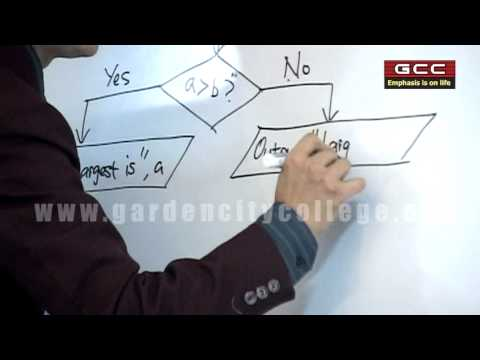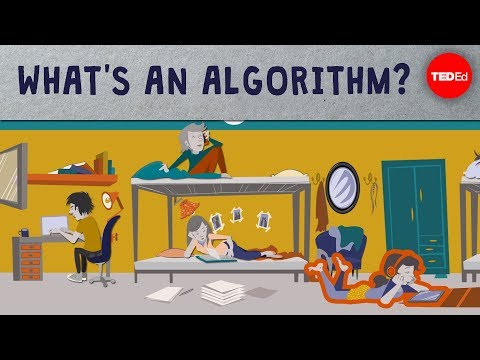## Algorithm

Subject: Computer Basics

Find Your Query

#### Overview

This note explains what alogorithm is.

#### Computer Program:

We know that computer is an user dependent machine. It doesn't work or think on its own. All the activities of computer system are controlled and processed by the instructions and information provided by the user. These instruction are called commands. Collection of such instruction and the data in a systematic form to solve the problem is called the program.

To solve the problem using computer we have to make some systematic plan of action. Such plan of action can be represented in the systematic order using different tools and technique called the program presentation tools and technique. Popular tools and technique used to represent the programs are flowchart and algorithm.

#### Algorithm

Algorithm is defined as a set of rules that define how a particular problem can be solved in finite number of steps. In another word, algorithm is a step by step representation of instructions to solve the problem. An algorithm must be composed of a finite set of steps, each of which may require one or more operations.

The good algorithm should have following features:

• Input: specified and required input values.
• Output: outcome values or solution of the problem.
• Definite: What should be done must be clearly defined.
• Effective: In finite amount of time it must be able to perform each step in finite amount.
• Finite: There must be finite number of steps or operations.
• Correct: Desirable and correct output must be able to generate.

#### Example of an algorithm

Problem: Find the largest number in an unsorted list of numbers

Let's say the input consists of a list of numbers, and this list is called L. The number L1 would be the first number in the list, L2 the second number, etc. And we know the list is not sorted - otherwise the answer would be really easy. So, the input to the algorithm is a list of numbers, and the output should be the largest number in the list.

Step 1: Let Largest = L1
Step 2: For each item in the list:
Step 3: If the item is greater than Largest:
Step 4: Then Largest = the item
Step 5: Return Largest

##### Things to remember
• Algorithm is defined as a set of rules that define how a particular problem can be solved in finite number of steps.
• It includes every relationship which established among the people.
• There can be more than one community in a society. Community smaller than society.
• It is a network of social relationships which cannot see or touched.
• common interests and common objectives are not necessary for society.
##### Videos for Algorithm##### Concepts of Algorithm, Flow Chart & C Programming##### Questions and Answers

An algorithm is a sequence of steps required to solve a particular problem. The concept of algorithm was given by ALKHOWARISMI.

1. Some characteristic of an algorithm are listed below:
2. Each and every instruction should be precise and unambiguous.
3. An algorithm should have finite number of steps.
4. An algorithm should produce correct result.
5. An algorithm should not use particular programming language.

lgorithm

Step 1 : Start

Step 2 : Read radius and store to R

Step 3 : Assign value of pie to P

Step 4 : Find area and circumference

Step 5 : Display area and circumference

Step 6 : StopAlgorithm.

Step 1 : Start

Step 2 : Read A, B

Step 3 : Assign A to X and B to Y

Step 4 : Divide A by B.

Step 5 : Is remainder zero? If yes, go to step 7.

Step 6 : Assign A to B and B to remainder. And go to step 4

Step 7 : Assign B to HCF

Step 8 : Multiply X and Y and divide by HCF. And assign result to LCM.

Step 9 : Display HCF and LCM

Step 10 : Stop##### Quiz

© 2019-20 Kullabs. All Rights Reserved.